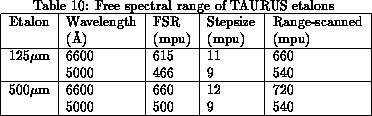Previous: Rotator PA
Up: Setting up
Previous Page: Rotator PA
Next Page: Observing

## Setting datacube parameters

In order to set up the datacube parameters it is necesssary to specify the detector format (i.e. the x and y dimensions and pixel size for the datacube) and the parameters for the etalon scanning (i.e. the z axis and pixel size for the datacube). The detector format used depends as usual on the required fieldsize and spatial resolution. The etalon scanning parameters depend on the free spectral range, finesse and zero point. In order to determine these parameters it is normally necessary to take a few trial exposures.

Since setting up TAURUS for observing is rather complex, ICL procedures have been produced to guide the observer through the process. These are described in section 15. Briefly, the FP_MODE procedure is used to specify the detector being used, and the FP_SETUP procedure is used to specify all the observing parameters. Subsets of the parameters can be altered using FP_SETUP_2D and CS100_TABLE. Finally, datacubes are acquired using FP_EXP_3D and images are acquired using FP_EXP_2D.

In order to specify the etalon scanning parameters, you will first need to determine the free spectral range (FSR) of the etalon at the wavelength of the observations. Note that the FSR is linearly proportional to the wavelength of the observations, so given an estimate at one wavelength it is straightforward to obtain an estimate at a different wavelength. The nominal FSR for the standard etalons at standard wavelengths are is given in Table 10. A log of actual measurements is stored on-line on the 8300 in the directory [TAURUS.INSTR.CS100]. The FSR is normally specified in microprocessor units (mpu).If you wish to measure the FSR yourself, this is done as follows using observations of an arc line. See section 16.1 for a summary of useful arc lines. First set up for data-acquisition using the FP_MODE and FP_SETUP commands. Then take a series of 2D exposures using FP_EXP_2D, altering the etalon gap each time using FP_SETUP_2D, until you can see a nice ring. Note the Z offset from the mimic. Keep increasing the gap, until the same ring reappears with the same radius. Note the Z offset again. The difference between the two values of the Z offset is the FSR. In order to be able to reduce the data, it is normally necessary to scan the etalon over slightly more than the FSR. For example, if the FSR is 600 microprocessor units, scan the etalon over 660 units.

The number of planes in the datacube is determined by the etalon finesse. The TAURUS etalons have a finesse of between 20 and 30, implying that about 60 planes are required in the datacube. Note that the instrumental profile of a Fabry-Perot interferometer is not Gaussian, but has rather more power in the wings. It is therefore advisable to oversample slightly. Thus in the example above, where the FSR is equal to 600, it would be normal to step the etalon 60 times, incrementing the gap by 11 microprocessor units each time.

Table 10 summarises suggested etalon stepsizes and scan ranges, assuming that the datacube has 60 planes.

The zero point of the etalon scan is determined primarily by the properties of the phase calibration cube (see section 16.1). It is usually preferable to set the zero-point such that the calibration line appears both at the start and end of the scan i.e. good data is obtained on the calibration line in two adjacent orders of interference.

Thu Apr 7 00:29:52 BST 1994